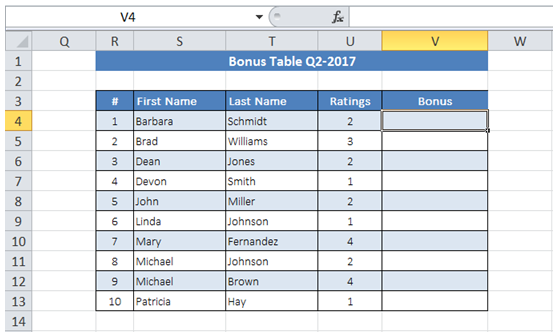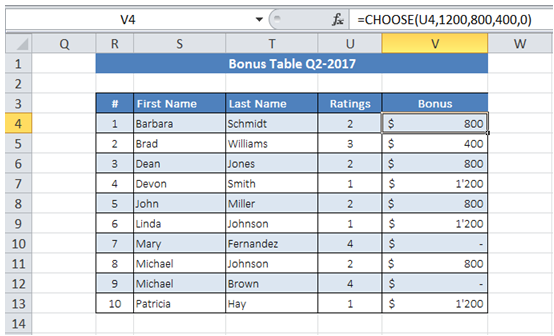## Rate staff for bonus in Excel

For example in the table below I have the ratings of all my staff. I would like to set up the table for automatic bonus calculation based on the ratings -

Ratings 1 - \$1200, 2 - \$800, 3 - \$400 and 4 - \$0.To do it in Excel, here is the answer:

a) Enter the formula =CHOOSE(U4,1200,800,400,0) in "Bonus" column for first employee. Drag the formula all the way down to end of column.

The first argument is the Index to choose from one among many values entered in the subsequent section of formula.

For example, for the first employee, rating is 2. Hence second value 800, from among the set of values (1200, 800, 400, 0) is selected by formula.